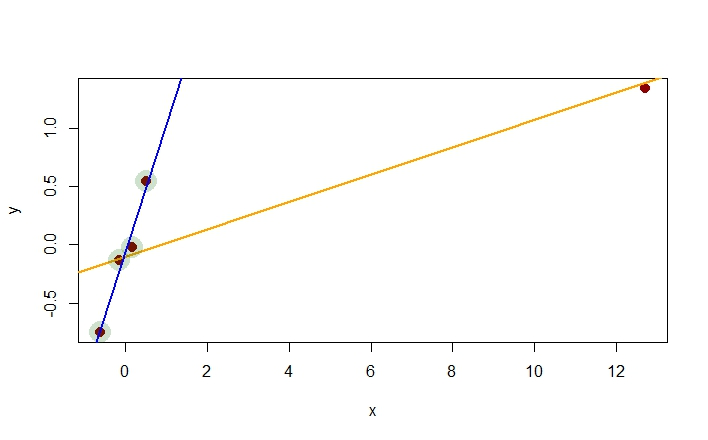### OLS DIAGNOSTICS:

First off, setting up a toy example, from the first rows of the dataset mtcars:

d = mtcars[1:6,]                          # The dataset
fit = lm(mpg ~ wt + disp, d)              # The OLS model
X = model.matrix(fit)                     # The model matrix X
H = X %*% (solve(t(X) %*% X) %*% t(X))    # The hat matrix
diagonal = hat(model.matrix(fit))         # The diagonal of the hat matrix

#### Dfbeta:

DFBETA measures the difference in each parameter estimate with and without a presumably influential point. There is a DFBETA for each point and each observation (if there are $$N$$ points and $$k$$ variables there are $$N\times k$$ DFBETAs).

# Let's look at the leverage of the fist entry in the database:
d[1,]
##           mpg cyl disp  hp drat   wt  qsec vs am gear carb
## Mazda RX4  21   6  160 110  3.9 2.62 16.46  0  1    4    4
# First calculate the coefficients fitting the model without this entry:
dno = d[-1,]
fitno = lm(mpg ~ wt + disp, dno)
coefficients(fit) - coefficients(fitno)
##   (Intercept)            wt          disp
## -1.5146662238  0.5115198590 -0.0008971436
# More expediently,
dfbeta(fit)[1,]
##   (Intercept)            wt          disp
## -1.5146662238  0.5115198590 -0.0008971436

#### Leverage or influence or hat value:

In statistics, the projection matrix , sometimes also called the influence matrix or hat matrix , maps the vector of response values (dependent variable values) to the vector of fitted values (or predicted values). It describes the influence each response value has on each fitted value.

1 - (residuals(fit) / (d[1,'mpg'] - predict(fitno, d[1,])))
## Mazda RX4
## 0.3009581
# In-built function:

hatvalues(fit)
## Mazda RX4
## 0.3009581
# Or find the value in the diagnonal of the hat matrix:

H
##  0.3009581

#### Standardized residuals:

First some definitions:

The deviance is the sum of squared residuals:

all.equal(deviance(fit), sum(residuals(fit)^2))
##  TRUE

The MSE (MEAN SQUARED ERROR) is defined as $$s^2=\frac{e^\top e}{n-p}$$ with $$\vec e$$ corresponding to the residuals, and $$\mathbf e^\top \mathbf e$$ given by the deviance; $$n,$$ the number of observations nrow(X); and $$p,$$ the number of parameters col(X), including the intersect.

MSE = deviance(fit)/ df.residual(fit)

# Notice that

all.equal(nrow(X) - ncol(X), df.residual(fit))
##  TRUE
# Standardized residuals:

fit$residuals / sqrt(MSE * (1 - hatvalues(fit))) ## Mazda RX4 Mazda RX4 Wag Datsun 710 Hornet 4 Drive ## -0.7710246 0.2446626 0.1153891 1.5970065 ## Hornet Sportabout Valiant ## -1.2633702 -1.1403043 # The built-in formula is rstandard(fit)  ## Mazda RX4 Mazda RX4 Wag Datsun 710 Hornet 4 Drive ## -0.7710246 0.2446626 0.1153891 1.5970065 ## Hornet Sportabout Valiant ## -1.2633702 -1.1403043 #### Studentized residuals: MSEno = deviance(fitno) / fitno$df.residual
resid(fit)/ sqrt(MSEno * (1 - hatvalues(fit)))
##  Mazda RX4
## -0.7030378
# or

rstudent(fit)
##  Mazda RX4
## -0.7030378

#### Cook’s distance:

Cook’s distance is the scaled change in fitted values. Each element in Cook’s distance is the normalized change in the vector of coefficients due to the deletion of an observation. The Cook’s distance, $$D_i,$$ of observation $$i$$ is

$D_i=\frac{\sum_{j=1}^N\,\left( \hat y_j-\hat y_{j(i)} \right)^2}{\text{MSE}\; p}$

where $$\hat y_j$$ is the $$j$$-th fitted response; $$\hat y_{j(i)}$$ is the $$j$$-th fitted response value when the coefficients are calculated without the observation $$i;$$ $$\text {MSE}$$ is the mean squared error; and $$p$$ is the number of coefficients, including the intercept.

dy = predict(fitno, d) - predict(fit, d)
sum(dy^2) / (ncol(X) * MSE)
##  0.08531356
# alternative formula:

fit$residuals^2 /(ncol(X)*sum(fit$residuals^2)/df.residual(fit))*(hatvalues(fit)/(1-hatvalues(fit))^2)
##  Mazda RX4
## 0.08531356
cooks.distance(fit)
##  Mazda RX4
## 0.08531356

This was an original question posted in CV.

I am trying to replicate what the function dfbetas() does in R.

dfbeta() is not an issue… Here is a set of vectors:

x <- c(0.512, 0.166, -0.142, -0.614, 12.72)
y <- c(0.545, -0.02, -0.137, -0.751, 1.344)

If I fit two regression models as follows:

fit1 <- lm(y ~ x)
fit2 <- lm(y[-5] ~ x[-5])

I see that eliminating the last point results in a very different slope (blue line - steeper):enter image description here

This is reflected in the change in slopes:

fit1$coeff - fit2$coeff
-0.9754245

which coincides with the dfbeta(fit1) for the fifth value.

Now if I want to standardize this change in slope (obtain dfbetas) and I resort to:

Williams, D. A. (1987) Generalized linear model diagnostics using the deviance and single case deletions. Applied Statistics 36, 181–191

which I think may be one of the references in the R documentation under the package {stats}. There the formula for dfbetas is:

$$\large dfbetas (i,fit) = \Large {(\hat{b} - \hat{b_{-i}})\over SE\, \hat{b_{-i}}}$$

This could be easily calculated in R:

(fit1$coef - fit2$coef)/summary(fit2)$coef yielding: -6.79799 The question is why I am not getting the fifth value for the slope in: dfbetas(fit1) (Intercept) x 1 1.06199661 -0.39123009 2 0.06925319 -0.02907481 3 -0.02165967 0.01003539 4 -1.24491242 0.65495527 5 -0.54223793 -93.81415653! What is the right equation to go from dfbeta to dfbetas? And this is the answer in CV: $$DFBETAS_{k(i)}$$ is calculated by: $$\Large \frac{b_k-b_{k(i)}}{\sqrt{MSE_{(i)}c_{kk}}}$$, for $$k$$ = 1, 2, . . . , $$p$$. $$p$$ is the number of regression parameters or coefficients. where $$b_k$$ is the $$k$$th regression coefficient that uses all the data and $$b_{k(i)}$$ is the same coefficient with the $$i$$th case deleted. $$MSE_{(i)}$$ here is the mean-square error from the regression where the $$i$$ case is deleted and $$c_{kk}$$ is the $$k$$th diagonal element of the unscaled covariance matrix $$(X^{\prime}X)^{-1}$$. So you can calculate $$DFBETAS_{k(i)}$$ manually with the following R code: numerator<-(fit1$coef - fit2$coef) denominator<-sqrt((summary(fit2)$sigma^2)*diag(summary(fit1)\$cov.unscaled))
DFBETAS<-numerator/denominator
DFBETAS
x
93.81416